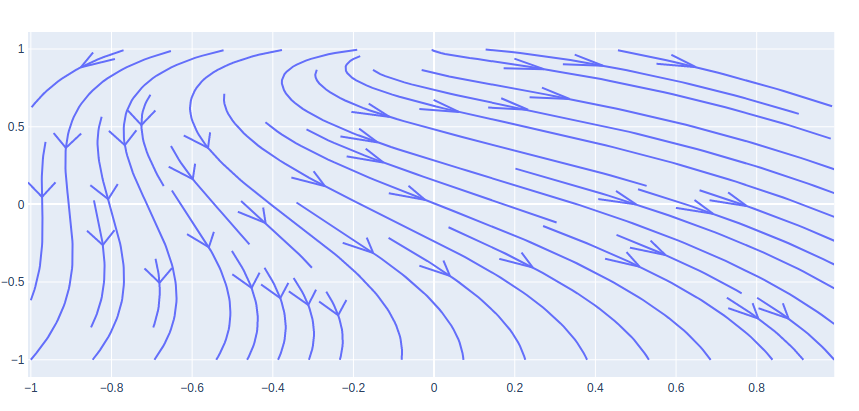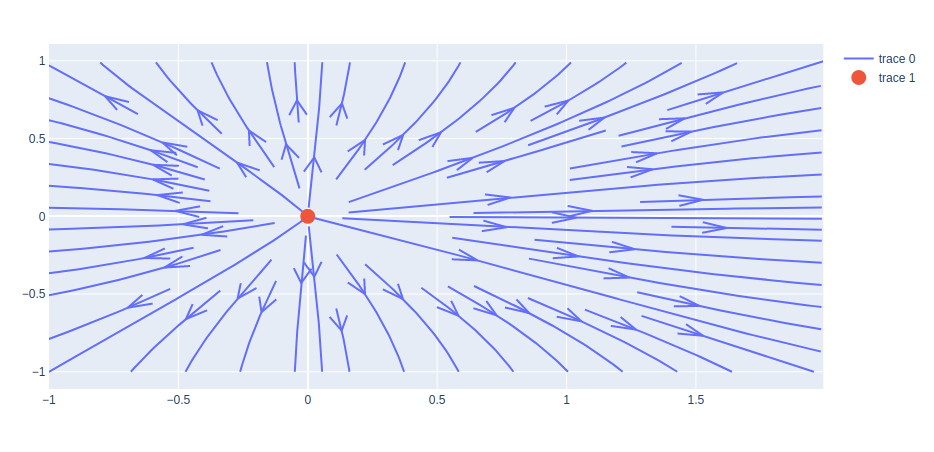GeeksforGeeks App
Open AppBrowser
Continue

# Streamline Plots in Plotly using Python

A Plotly is a Python library that is used to design graphs, especially interactive graphs. It can plot various graphs and charts like histogram, barplot, boxplot, spreadplot, and many more. It is mainly used in data analysis as well as financial analysis. plotly is an interactive visualization library.

## Streamline Plots in Plotly

In plotly, streamline plots are based on the representation on a 2-D vector field which is explained as velocity fields, which are consist of closed curves that are tangent to the velocity field. Streamlining is the fastest technique and more efficient for getting the data. Velocity values are interpolated when determining the streamlines. Streamlines are initialized on the boundary of the x-y domain.

Syntax: create_streamline(x, y, u, v, density=1, angle=0.3490658503988659, arrow_scale=0.09)

Parameters:

x: 1 dimensional, evenly spaced list or array

y: 1 dimensional, evenly spaced list or array

u: 2 dimensional array

v: 2 dimensional array

density: controls the density of streamlines in plot. This is multiplied by 30 to scale similarly to other available streamline functions such as matplotlib. Default = 1

angle: angle of arrowhead. Default = pi/9

arrow_scale: value to scale length of arrowhead Default = .09

Example:

## Python3

 `import` `plotly.figure_factory as ff``import` `numpy as np` `x ``=` `np.linspace(``-``1``, ``1``, ``10``)``y ``=` `np.linspace(``-``1``, ``1``, ``10``)``Y, X ``=` `np.meshgrid(x, y)``u ``=` `1` `-` `X``*``*``2` `+` `Y``v ``=` `-``1` `+` `X ``-` `Y``*``*``2` `# Create streamline figure``fig ``=` `ff.create_streamline(x, y, u, v, arrow_scale``=``.``1``)``fig.show()`

Output:## Plotting the Source Point

The source point can be plot using the scatterplot() method of the graph_objects class. In this, the streamline plot and the position of the marker in the scatterplot are tweaked in such a way that it seems that all the stream is originating from a particular source.

Example:

## Python3

 `import` `plotly.figure_factory as ff``import` `plotly.graph_objects as go``import` `numpy as np` `x ``=` `np.linspace(``-``1``, ``2``, ``50``)``y ``=` `np.linspace(``-``1``, ``1``, ``50``)``Y, X ``=` `np.meshgrid(x, y)``u ``=` `np.cos(X)``*``Y``v ``=` `np.cos(y)``*``X` `# Source for x and y coordinate``# of scatter plot``X, Y ``=` `0``, ``0` `# Create streamline figure``fig ``=` `ff.create_streamline(x, y, u, v, arrow_scale``=``.``1``)` `fig.add_trace(go.Scatter(x``=``[X], y``=``[Y],``                          ``mode``=``'markers'``,``                          ``marker_size``=``15``,``                        ``))` `fig.show()`

Output:My Personal Notes arrow_drop_up